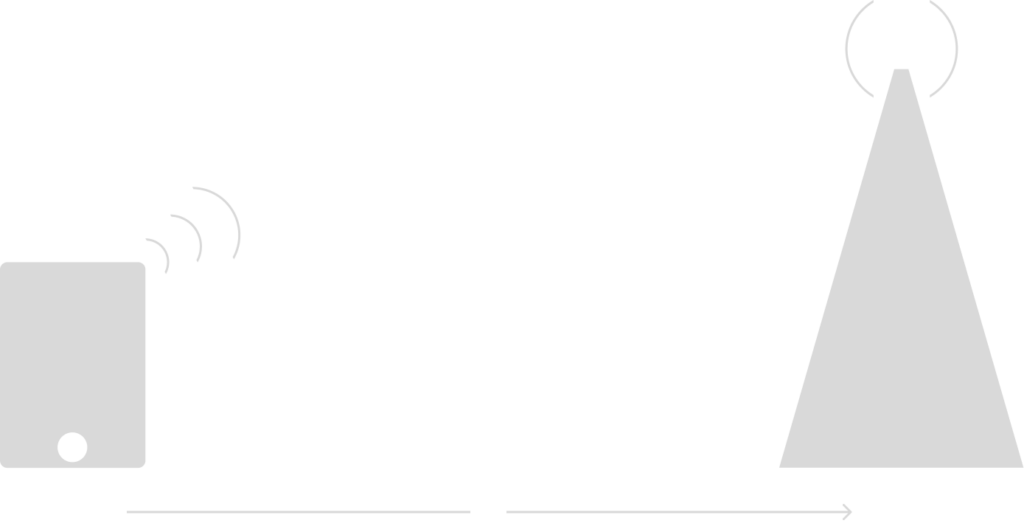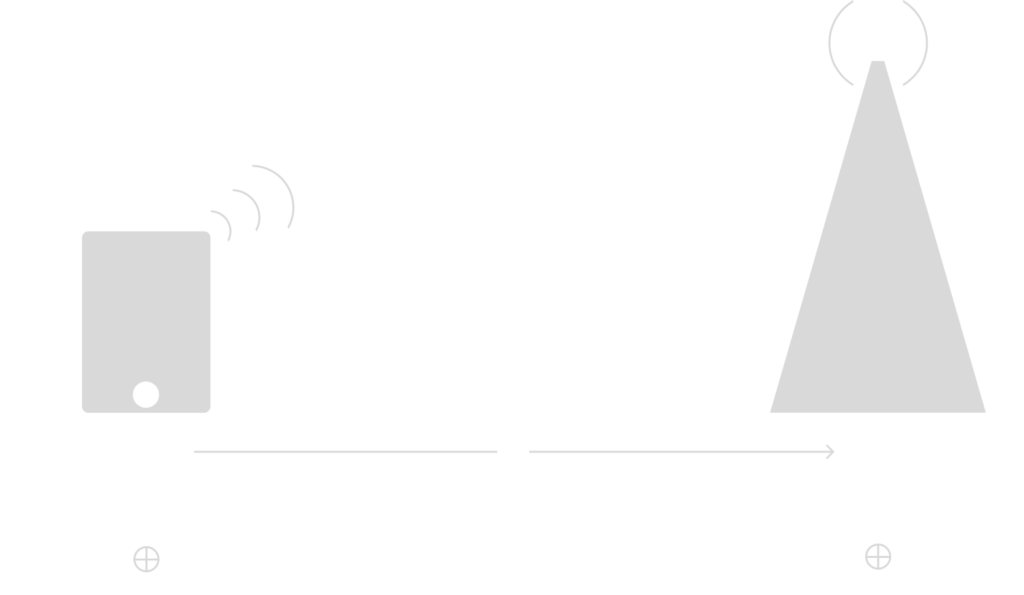Back To All

# Stream Ciphers, Random Numbers, and the One-Time PadSymmetric cryptography is split into two types, stream ciphers, and block ciphers. Stream ciphers encrypt one bit at a time and block ciphers encrypt multiple bits at a time. This post is dedicated to stream ciphers, if you want to learn about block ciphers make sure to read my DES and AES posts.

## Stream Ciphers

For some motivation, one of the first consumer uses of cryptography was inside mobile phones. They use a stream cipher to encrypt your voice that gets sent to a hub and then is decrypted before being sent to the receiver.### Definition

Stream ciphers encrypt bits individually. This is achieved by adding a bit from a key stream to a plaintext bit.
Definition from “Understanding Cryptography” by Christof Paar

### Algorithm

Let xi, yi, si ∈ ℤ2
Encryption: yi = e(xi) ≡ xi + si mod 2
Decryption: xi = d(yi) ≡ yi + si mod 2
Where i is an index to indicate which bit we are working on.

You may ask “Why are the encryption and decryption the same?”, especially if you are reading this after my shift cipher post, these may look similar but decryption used subtraction in the shift cipher.
The reason encryption and decryption are the same function can be shown easily below:

d(yi) ≡ yi + si mod 2
≡ (xi + si) + si mod 2
≡ xi + 2 ⋅ si mod 2  (Note: we are in mod 2, so 2 ≡ 0 mod 2)
≡ xi mod 2

The takeaway is that in mod 2 addition and subtraction are the same operations. This should be familiar to you if you have ever learned the truth table for the XOR gate. If you have not don’t worry I’ll show it below, but this is an important fact: Modulo 2 addition is equivalent to the XOR operation.

##### XOR table
 xi si yi 0 0 0 0 1 1 1 0 1 1 1 0Notice a nice cryptographic property of this, if you are talking on the phone and it needs to encrypt your 0 bit, then the cipher can use 0 or 1 for the keystream, and if it uses 0 your 0 becomes a 0, and if it uses 1 your 0 becomes a 1. The same thing if you need to encrypt a 1 bit. So it’s important to state: Any plaintext bit can be encrypted to either 0 or 1.
Thus if we can randomly choose our key stream to choose a 0 or 1 to be 50/50 chance, we actually have a pretty secure and simple. cipher

### Example

Let’s encrypt the ASCII character ‘A’:
x7 … x1 = 1000001
s7 … s1 = + 0101101   (This is the ASCII value for “l”)
y7 … y1 = 1101100
Now let’s decrypt:
y7 … y1 = 1101100
s7 … s1 = + 0101101
= 1000001 = “A”

### Conclusion

You may be thinking, “Wow, the stream cipher is very simple and I said it was pretty secure so why isn’t this used for everything?”. I’ll be honest, I downplayed just how hard it actually is to get the keystream, and it’s a whole other problem to make sure it’s cryptographically secure. That is what the next section will cover.
The next section will go over how to generate the key stream randomly, while also making sure Malcolm (our man in the middle) can not easily decrypt our message as well.

## Random Number Generators (RNGs)

There are three main families to distinguish between:
a) True Random Number Generators (TRNG)
b) Pseudo Random Number Generarots (PRNG)
c) Cryptographically secure Pseudo Random Number Generators (CPRNG)

### True Random Number Generators (TRNG)

A TRNG is just as the name suggests, they are truly random. These are often based on random physical processes.
For example s coin flip, dice, and Roulette. Usually, we want to use a computer, so some techniques to get randomness from a computer could be getting the mouse position coordinates, a person’s typing speed, or even the heat of a processor. These are all relatively random processes and are used to get truly random numbers.

TRNG is great but does not suit our needs in cryptography since we need to be able to share the key stream with another person. So if we did use a TRNG to get our key stream both parties would almost definitely get a different key stream. Therefore, we need something that can be deterministic.

### Pseudo Random Number Generators (PRNG)

A PRNG is computed, ie they are deterministic. They all often have a similar recursive algorithm to compute pseudo-random numbers in the following way:
si = seed
si + 1 = f(si), i = 0, 1, 2, …
For example here is the rand() function in C which is a pseudo-random number generator:
s0 = 12345
si + 1 = 1103515245si + 12345 mod 231 , i = 0, 1, 2, …

PRNGs seem pretty suitable and are deterministic. Which I said is what we need, but they are still unusable. If we wanted to use it in a cryptosystem we need to make sure our attacker can’t also generate the PRNG.

### Cryptographically Secure Pseudo Random Number Generators (CPRNG)

CPRNGs are PRNGs with an additional property: the numbers are unpredictable. Informally this means that given n output bits of the keystream, where n is some integer, it is computationally infeasible to compute the next bits.
A more precise definition is that given n consecutive bits of the keystream, there is no polynomial time algorithm that can predict the next bit with a better than 50% chance of success.
Definition from “Understanding Cryptography” by Christof Paar

The goal of this is to build a “perfect” cipher. So let’s define what we mean by a “perfect” cipher.
Def: A cipher is “Unconditionally secure” if it cannot be broken even with infinite computing resources.
The important word in this is infinite, its a little weird to wrap your head around so just think of an example of a cipher with a key space of |k| = 21000. This is computationally impossible to break because we just don’t have enough computers, remember that even a cipher with |k| = 2256 is considered secure. But it is not “unconditionally secure”, because if we somehow did have 21000 computers, each trying 1 key a second, the cipher could be broken in a second.
But let’s go ahead and create this “unconditionally secure” cipher below.

### Definition

The OTP is a stream cipher where:
1) The keystream bits si stem from a TRNG.
2) Each keystream bit is used only once.

Interestingly enough this simple cipher cannot be broken ever. The OTP is an “Unconditionally secure” cipher that an infinite amount of computers could never break.
But you always need to think about implementation, even though a cipher may be the most secure doesn’t mean it is practical. Remember to use this cipher you must also share the keystream, we said before that sharing a TRNG-generated keystream is next to impossible.

### Drawbacks

The key is as long as the message. For example, imagine you are trying to share a 400 MB encrypted file with someone using an OTP. You now also need to share the key, so now you just doubled the size of the file you just shared. On top of that, you also have to remember that you need to keep this keystream a secret from attackers.
Another drawback is that you can only use the keystream once, or else they become unsecure. If you don’t believe me, ask a spy for Pakistan that used OTP more than once and was caught.